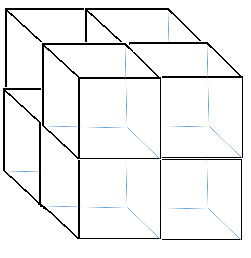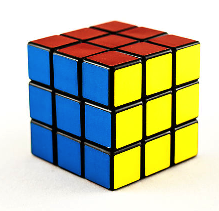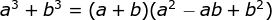# How to Factor a Perfect Cube: Formula & Examples

An error occurred trying to load this video.

Try refreshing the page, or contact customer support.

Coming up next: Using Tables and Graphs in the Real World

### You're on a roll. Keep up the good work!

Replay
Your next lesson will play in 10 seconds
• 0:02 Definitions
• 1:37 Factoring a Perfect…
• 3:09 Examples
• 8:51 Lesson Summary

Want to watch this again later?

Timeline
Autoplay
Autoplay
Speed

#### Recommended Lessons and Courses for You

Lesson Transcript
Instructor: Miriam Snare

Miriam has taught middle- and high-school math for over 10 years and has a master's degree in Curriculum and Instruction.

In this lesson, you will learn how to factor sums and differences of perfect cube binomials. You will learn to use the formula that gives you the factors through a few examples.

## Definitions

A perfect cube is any expression that can represent the volume of a cube. Imagine reaching into a toy bin and pulling out several building blocks and then try to arrange the blocks into a cube. Let's say you pulled out 4 blocks. You can make a square of 2 blocks by 2 blocks with 4 blocks, but it will only have a height of 1 block, so that does not make a cube. In order to create a cube, you would need to pull out 4 more blocks to layer on top of the square you made, like this:Now, you have a volume of 8 blocks that make a cube whose length, width, and height are all 2. So, 8 is a perfect cube number because 2 x 2 x 2 = 8 or 2^3 = 8.

Say you wanted to increase the size of the cube to be 3 blocks along each side, to look like this:Then, you would need 19 more blocks for a total of 27, because 3 x 3 x 3 = 3^3 = 27.

All of the perfect cubes result from raising an integer, a whole number or its negative counterpart, or a variable to the third power. These expressions are called cubes because the volume of a cube is found by raising the length of its side to the third power.

A perfect cube binomial is an expression consisting of two terms that are perfect cubes. These terms are either added or subtracted from each other.

## Factoring a Perfect Cube Binomial

Factors are expressions that are multiplied together. So, factoring asks you to break down an expression into more simple expressions that are multiplied. The factors of a perfect cube binomial may not look very simple because they end up being a binomial, two terms added or subtracted, times a trinomial, three terms added or subtracted. However, there is a formula that gives you the factors quite easily.

When faced with assorted factoring problems, you have different methods to tackle different expressions. Make sure that you are applying the correct strategy for each type of problem. A perfect cube binomial is fairly easy to identify, and it can always be factored using this formula. Your first clues will be that there are always two terms and the variables have exponents that are multiples of 3. Once you have spotted those characteristics, you want to check that the coefficient (number multiplied to variables) and constant (number with no variables) of both terms are perfect cube numbers.

Once you are sure that you are factoring a perfect cube binomial, you are going to use this formula:Here's a hint: If you have a difference of cubes in the form a^3 - b^3, just think of it as a^3 + (-b^3). That way you only have to memorize this one formula.

## Examples

Let's take a look at some examples:

#### Example 1

Factor: x^3 + 64

In this problem, we see that two terms have been added together, making it a binomial. Both of the terms are perfect cubes. We can tell that from the power of 3 on the x, and we know 64 is a perfect cube because a cube measuring 4 x 4 x 4 has a volume of 4^3 = 64. To factor the expression, we do the following:

1. We determine what the a and b for our formula are. The first term is easy, x is the a. In the second term, 64 = 4^3, so b is 4.
2. Then, we substitute those values into the formula: (x + 4)(x^2 - (x)(4) + 4^2).
3. We can rewrite the (x)(4) as 4x, and evaluate the exponent in the last term to clean it up. Our final factored form is: (x + 4)(x^2 - 4x + 16).

#### Example 2

Here's another example. Factor: 8x^3 - 125

In this problem, we see that two terms subtracted from each other, making it a binomial. The power of 3 on the x tells us that we are most likely dealing with a perfect cube binomial. Let's check the numbers to be sure they are perfect cubes. The number 8 is a perfect cube because, as we discussed at the beginning of this lesson, 2^3 = 8. The number 125 is a perfect cube because a cube measuring 5 x 5 x 5 would have a volume of 5^3 = 125.

To factor the expression, we do the following:

To unlock this lesson you must be a Study.com Member.

### Register to view this lesson

Are you a student or a teacher?

### Unlock Your Education

#### See for yourself why 30 million people use Study.com

##### Become a Study.com member and start learning now.
Back
What teachers are saying about Study.com

### Earning College Credit

Did you know… We have over 160 college courses that prepare you to earn credit by exam that is accepted by over 1,500 colleges and universities. You can test out of the first two years of college and save thousands off your degree. Anyone can earn credit-by-exam regardless of age or education level.